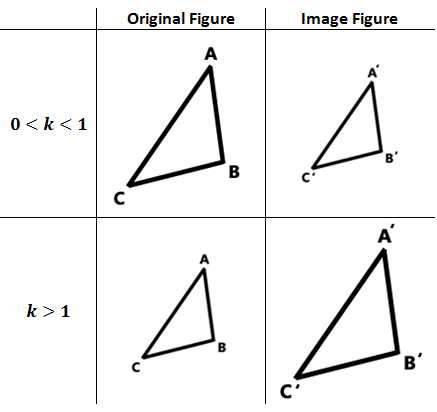Dilations
 Home > Lessons > Dilations Search | Updated December 22nd, 2018
Introduction
In this section of MATHguide, you will learn about dilations. Here are the topics within this page:

 Characteristics Similar Figures -- a topic covered in another section -- can be described by the mathematics of dilations.     Dilations have these features: They have a center of dilation. They have a scale factor. There is the original figure and it's image figure. Labeling Dilations When we refer to the original figure, of say a triangle, we call the original figure △ABC. We call the image figure △A'B'C'. The second triangle is pronounced, "triangle A-prime, B-prime, C-prime." Scale Factor A characteristic called scale factor informs us of the relationship between the original figure and its image. The scale factor is typically called the k-value.     When the k-value is 0 < k < 1 [greater than zero but less than one], the image figure is smaller than the original figure. When the k-value is k > 1 [greater than one], the image figure is greater than the original figure. This information is encapsulated within the diagram that follows.Instructional Videos The video below will demonstrate exactly how dilations work.ideo: Dilations Interactive Quizzes Here is a quiz that can be used to test your understanding of dilations.uiz: Dilations Related Lessons View these related lessons.esson: Reducing Fractionsesson: Geometry Proofsesson: Proportionsesson: Similar Figuresesson: Areas and Lengths of Similar Figuresesson: Volumes and Lengths of Similar Solids